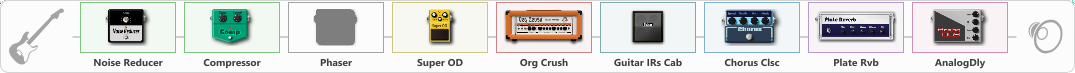# echo

Discussion in 'ToneLib-GFX presets' started by truss van halen, Jul 27, 2020.

1. ### truss van halenWell-Known Member

echo

Preset name: mirror slip heavy

Effects chain:Effect: "Noise Reducer" (Dynamics / Filter), active - "yes"
{
"Sens" = 66
"Mode" = Hard
}

Effect: "Compressor" (Dynamics / Filter), active - "yes"
{
"Sense" = 45
"Level" = 2
}

Effect: "Phaser" (Modulation / Sfx), active - "no"
{
"Model" = MXR Phase 90
"Speed" = 2.8
"Depth" = 68
"Reso" = 44
"Freq" = 6.2
}

Effect: "Super OD" (Overdrive / Distortion), active - "yes"
{
"Drive" = 50
"Tone" = 80
"Level" = 91
}

Effect: "Org Crush" (Amp simulators), active - "yes"
{
"Gain" = 46
"Bass" = 56
"Middle" = 67
"Treble" = 63
"Presence" = 50
"Master" = 76
"Level (dB)" = -4
}

Effect: "Guitar IRs Cab" (Cabinets), active - "yes"
{
"Model" = Orange PPC (4x12")
"Mic Position" = Edge
"Mic Distance" = Far
"Low Cut (Hz)" = 127
"Hi Cut (kHz)" = 5.5
"Mix" = 100
"Level (dB)" = 7
}

Effect: "Chorus Clsc" (Modulation / Sfx), active - "yes"
{
"Speed" = 0.1
"Depth" = 0
"Center" = 1.0
"Mode" = Stereo
}

Effect: "Plate Rvb" (Reverb), active - "yes"
{
"Time" = 3.2
"PreDelay" = 2
"LoDamp" = 17
"HiDamp" = 50
"Mix" = 40
}

Effect: "AnalogDly" (Delay), active - "yes"
{
"Time" = 380
"Feedback" = 34
"Tone" = 65
"Mix" = 65
}

Note: You will need to download and install the ToneLib-GFX software to use the preset.

File size:
906 bytes
Views:
2,789